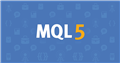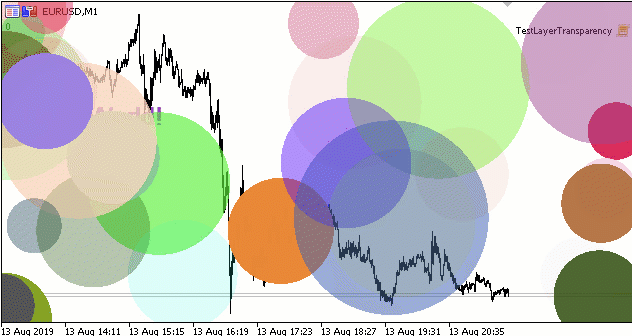# is there any plan for metatrader6?

i like metatrader but in ui other platform are much better. for example metatrader doens't support opacity colors or it has lack of objects ex: i need more fibonacci object like in motive wave.

https://www.mql5.com/en/docs/standardlibrary/canvasgraphics/ccanvas/ccanvastransparentlevelset

As of the rest : motivewave fibonacci trading platform is a payed for platform. Doesn't it tell it all?Documentation on MQL5: Standard Library / Custom Graphics / CCanvas / TransparentLevelSet
• www.mql5.com
Standard Library / Custom Graphics / CCanvas / TransparentLevelSet - Reference on algorithmic/automated trading language for MetaTrader 5

Too bad — you got what you paid for. It took 15 years to get OOP and non-forex. Wait some more and you might get your additions.

Mahdi Khoshroo:
i like metatrader but in ui other platform are much better. for example metatrader doens't support opacity colors or it has lack of objects ex: i need more fibonacci object like in motive wave.

I don't know what fibonacci has special in MotiveWave but I strongly doubt it can't be coded in MT5.

Of course it's not provided as standard with the platform, but MT5 provides all the basis to code almost anything.

Alain Verleyen:

I don't know what fibonacci has special in MotiveWave but I strongly doubt it can't be coded in MT5.

Of course it's not provided as standard with the platform, but MT5 provides all the basis to code almost anything.

it's not about kind of tools. my issue is about ui and ux

for example in motive wave the ui is awsome and you cand do anything without code ex: scan symbols to find signals without code

Mahdi Khoshroo:

it's not about kind of tools. my issue is about ui and ux

for example in motive wave the ui is awsome and you cand do anything without code ex: scan symbols to find signals without code

So what ?

MT5 is different. It provides the base tools, and allow to build almost all you want by code. It will not change for sure.

A new version only for a new UI and UX?

I can trade via command console... lol

Mahdi Khoshroo:
i like metatrader but in ui other platform are much better. for example metatrader doens't support opacity colors or it has lack of objects ex: i need more fibonacci object like in motive wave.

Форум по трейдингу, автоматическим торговым системам и тестированию торговых стратегий

Canvas - это круто!

Nikolai Semko, 2019.08.13 20:48

If canvas with transparency (COLOR_FORMAT_ARGB_NORMALIZE) is used, then the transparency of the two layers tr1 and tr2 should be mixed according to the formula

`double tr=tr1+tr2-tr1*tr2; // где tr, tr1, tr1 меняются от нуля (абсолютная прозрачность) до 1(абсолютная непрозрачность) `

An example script with random circles of random color and random transparency:```#include <Canvas\iCanvas.mqh> //https://www.mql5.com/ru/code/22164
#define Num 100
//+------------------------------------------------------------------+
//| Script program start function                                    |
//+------------------------------------------------------------------+
void OnStart()
{
// инициализация случайных окружностей
uint X[Num],Y[Num],sr[Num],clr[Num],Rmin[Num],Rmax[Num];
double sx[Num],sy[Num];
for(int i=0; i<Num; i++)
{
X[i]=rand()%3141;
Y[i]=rand()%3141;
sr[i]=rand()%3141;
sx[i]=0.3+0.7*(double)rand()/32767.0;
sy[i]=0.3+0.7*(double)rand()/32767.0;
clr[i]=ARGB(rand()%256,rand()%256,rand()%256,rand()%256);
int r2=5+rand()%100;
int r1=5+rand()%100;
Rmin[i]=(int)fmin(r1,r2);
Rmax[i]=(int)fmax(r1,r2);
}
// формирование изображения из Num (= 100) полупрозрачных кругов
double j=0;
while(!IsStopped())
{
Canvas.Erase();
Canvas.CurentFont("Arial",30);
Canvas.TextPosY=100;
Canvas.Comm("Hello World!");
for(int i=0; i<Num; i++)
{
Circle(W.Width/2*(1+sin((X[i]+sx[i]*j)*M_PI/1000)),
W.Height/2*(1+sin((Y[i]+sy[i]*j)*M_PI/1000)),
Rmin[i]+double(Rmax[i]-Rmin[i])/2*(1.0+cos((j+sr[i])*M_PI/1000)),clr[i]);
}
Canvas.Update();
j+=0.3;
Sleep(30);
}
}
//+------------------------------------------------------------------+

void Circle(double x,double y,double r,uint clr)
{
double R2=r*r;
for(int X=Round(x-r); X <=Round(x+r);X++)
for(int Y=Round(y-r); Y<=Round(y+r);Y++)
if(((x-X)*(x-X)+(y-Y)*(y-Y))<=R2) Canvas.PixelSet(X,Y,MixColor(clr,Canvas.PixelGet(X,Y)));
}
//+------------------------------------------------------------------+

uint MixColor(uint clr,uint clrback)// смешиваем цвет и прозрачность clr c цветом и прозрачностью фона clrback
{
argb C,Bg;
if(clrback==0) return clr;
C.clr=clr;
if(C.c==255) return clr;
Bg.clr=clrback;
double tr=C.c/255.0;
double trb=Bg.c/255.0;

C.c=uchar(Bg.c+tr*(C.c-Bg.c));
C.c=uchar(Bg.c+tr*(C.c-Bg.c));
C.c=uchar(Bg.c+tr*(C.c-Bg.c));

C.c=uchar((trb+tr-trb*tr)*255.0+0.49999);
return C.clr;
}
//+------------------------------------------------------------------+```
Check out this thread. You may be surprised at the graphic potential of MT5.

Did we even start at Metatrader 1 ? I have always wondered.

Tonny Obare:
Did we even start at Metatrader 1 ? I have always wondered.
I started with Metatrader 3 with Pentium I and Windows '95.
Windows '95 is the best Windows.